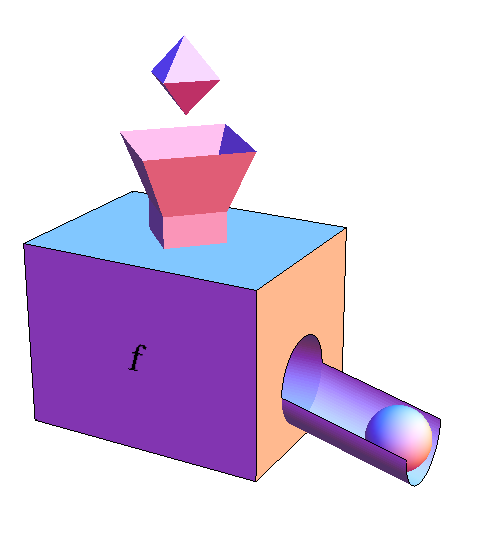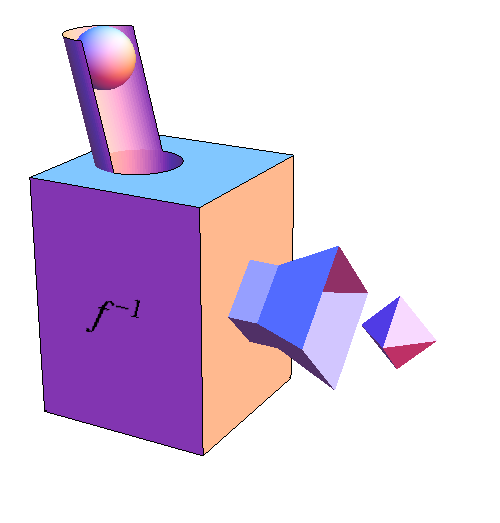# Math Insight

### The function machine inverse

If $f$ is a function, we can represent it by a function machine that can take any input from its domain and transform it to an element from its codomain. In this illustration, we represent elements of the domain of $f$ by octahedra and elements of its codomain by spheres. An octahedron enters through the function machine's input funnel, and the function machine spits out a particular sphere, where the identity of the sphere depends on which octahedron went in.Now, imagine that for each unique octahedron, the function machine yielded a different sphere. Then, by examining the sphere that came out of the function machine's chute, it would be possible to determine which octahedron went into the funnel. (We'd be in trouble if multiple distinct octahedron yielded the same sphere, as there would be no way to distinguish which octahedron went into the funnel by examining the sphere.)

In the case where we can examine the sphere and determine which octahedron went in, it makes sense that we could run $f$'s function machine backwards. We could put the sphere back in, and the backwards function machine could undo whatever $f$'s function machine did to produce the sphere. Then, the octahedron that started it all would pop out of the funnel.

To show that we are running the machine backwards, let's flip it over so that the output chute is at the top. This backwards machine takes in spheres into the chute and spits out octahedron out of the funnel. Since this backwards machine undoes whatever processing $f$'s function machine did, we call it the inverse of $f$, or simply “$f$ inverse.” We give $f$ inverse a special symbol, too: $f^{-1}$. The superscript here does not denote exponentiation; it simply indicates we are running the function machine backwards to undo the processing $f$ did.If we denote a particular octahedron by the variable $x$, then we can denote by $f(x)$ the resulting sphere that $f$'s function machine spits out. Or, to make it shorter, we could just use the variable $y$, where $y=f(x)$. By the definition of $f^{-1}$, if we stick the sphere $y$ into the $f^{-1}$ backwards function machine, we must get the octahedron $x$ back, i.e., $f^{-1}(y)=x$. If we composed $f$ and $f^{-1}$ by connecting their function machines, then the resulting composition would not do anything to the octahedron $x$. We would put the octahedron in the combined function machine and the same octahedron would come out. In math symbols, we would find that $f^{-1}(f(x))=x$, which we could also write as $(f^{-1} \circ f)(x)=x$.

You can read some examples of calculating the inverse function.